Get a head full of numbers in this quiz!

# Algebra Intermediate - Factoring Polynomials [Step 1: Greatest Common Factor]

This Math quiz is called 'Algebra Intermediate - Factoring Polynomials [Step 1: Greatest Common Factor]' and it has been written by teachers to help you if you are studying the subject at middle school. Playing educational quizzes is a fabulous way to learn if you are in the 6th, 7th or 8th grade - aged 11 to 14.

It costs only \$12.50 per month to play this quiz and over 3,500 others that help you with your school work. You can subscribe on the page at Join Us

A monomial refers to one term or one number. “10” is a monomial (mono stands for “1”) as it is one term. Now if you have a problem that has two monomials in a math equation such as: 10 + 5, both “10” and “5” are monomials but since they both appear in a math equation (shown by the addition symbol) they are together referred to as binomials (bi stands for “2”). When you have three monomials linked together in a math equation such as: 10 + 5 + 7, each by itself is a monomial but together in the equation they are referred to as a “trinomial” (tri stands for “3”).

When you have a string of four or more monomials linked in a mathematical equation such as: 10 + 5 + 7 - 6, then you have a polynomial (poly stands for “many”). However, binomials and trinomials can also be referred as a polynomial as “many” can refer to 2 or more. So, if you ever see a problem with two monomials and it is referred to as a polynomial, that reference is not wrong, just someone’s own preference in what to call it.

Okay, now that the quick review of pre-algebra is behind us, let’s move on to intermediate algebra and learning how to factor polynomials. When factoring polynomials, the first step that needs to be taken is to find the greatest common factor. Once you find the greatest common factor, the second step is to count the number of terms. From there you will need to address the situation where there are two terms (binomials), three terms (trinomials) and four terms (polynomials).

Hint: Whenever you see the word “factor” or “factoring” it tells you right away that you will be multiplying. So if you were asked what are the “factors” of the number 10 your answer would be: 1 x 10 = 10 and 2 x 5 = 10. In other words, when you are asked what are the factors of the number 10, you are asked to list all combinations that when multiplied together will give you the answer 10. With the number 10, there are only two factors, 1 x 10 and 2 x 5.

For this quiz on factoring polynomials, we will only be addressing the first step so that you can grasp what you must first do to solve a factoring polynomial problem or expression. So now let’s look at Step 1 below. The other steps will be addressed in separate quizzes.

Go straight to Quiz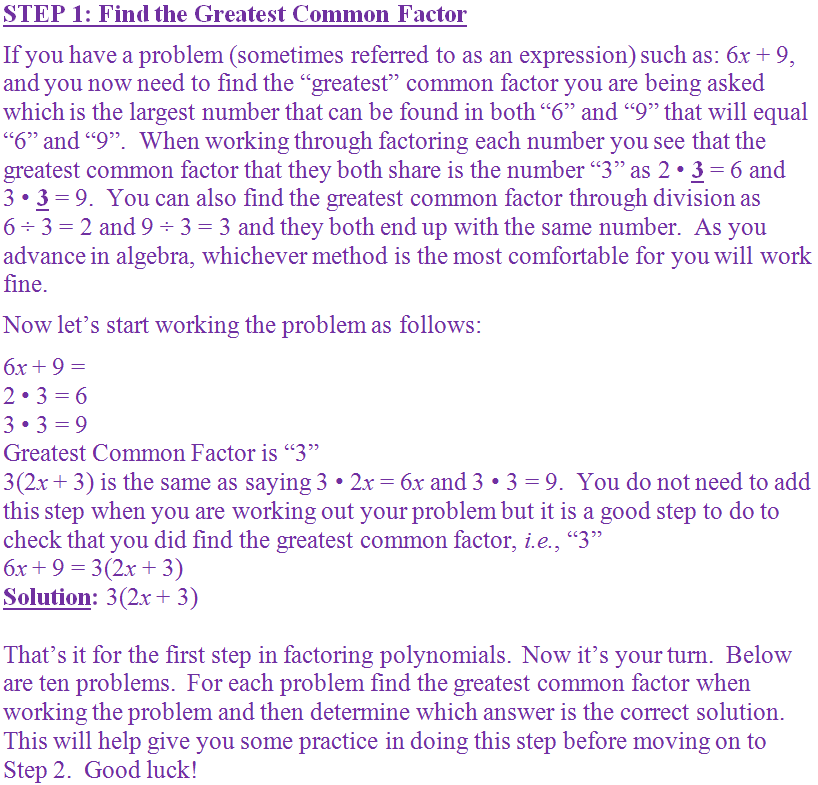1.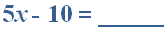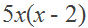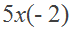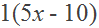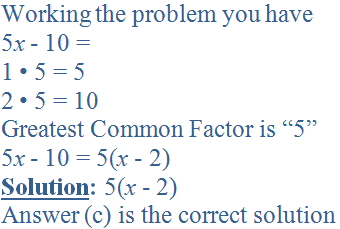2.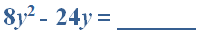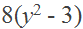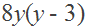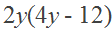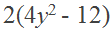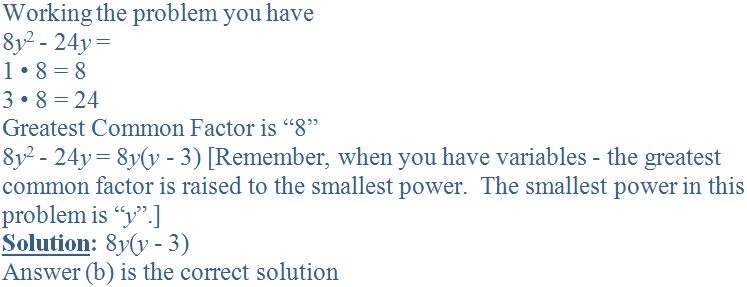3.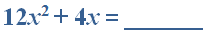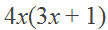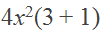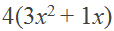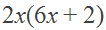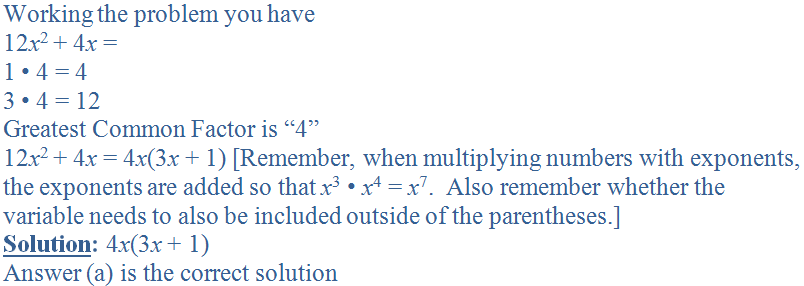4.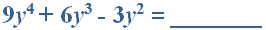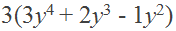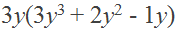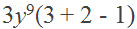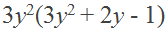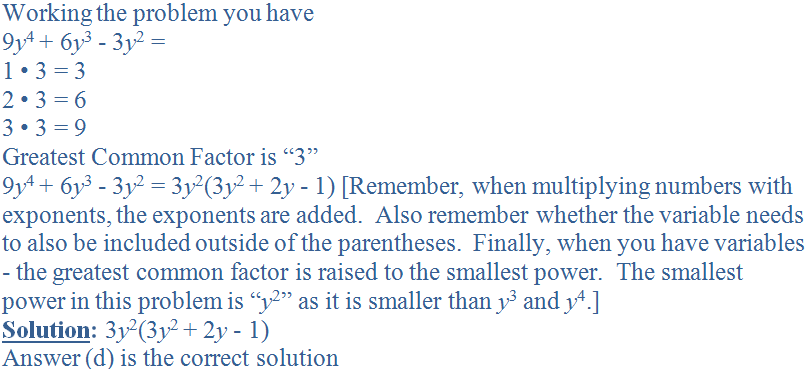5.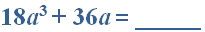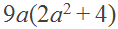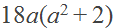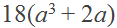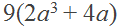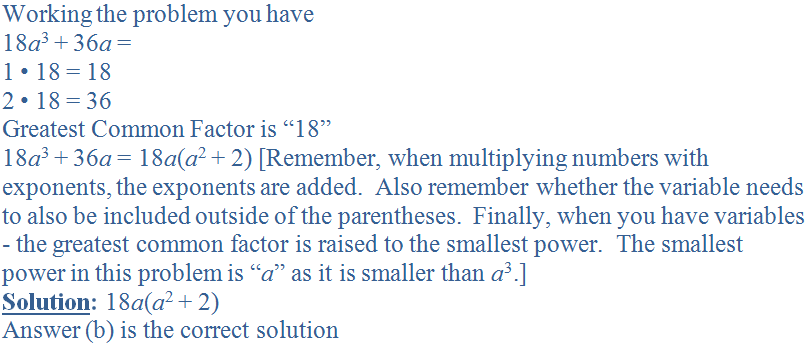6.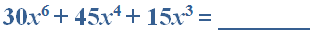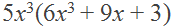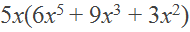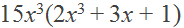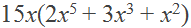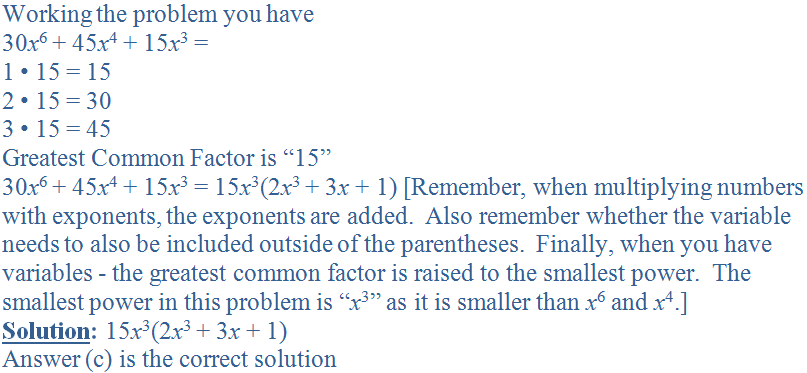7.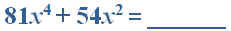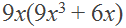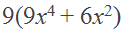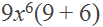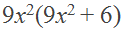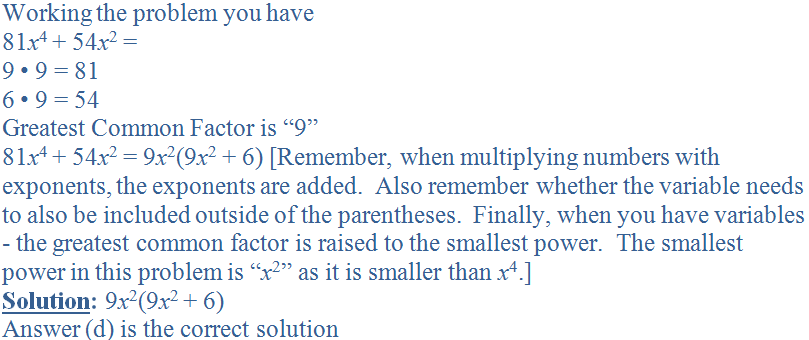8.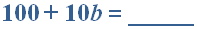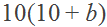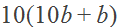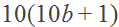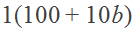9.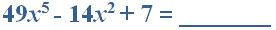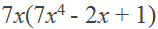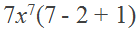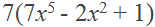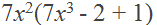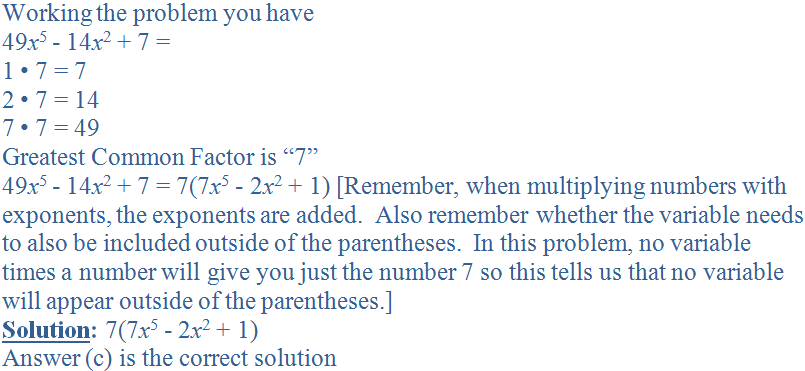10.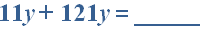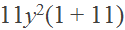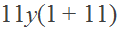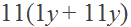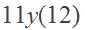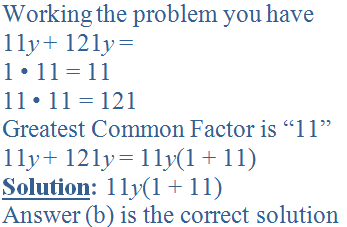Author:  Christine G. Broome